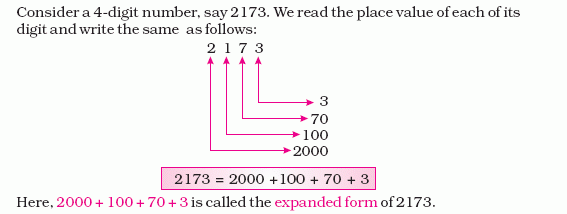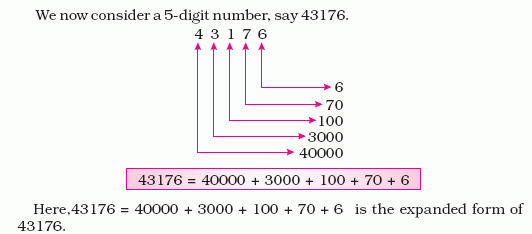Name: ___________________Date:___________________

 Email us to get an instant 20% discount on highly effective K-12 Math & English kwizNET Programs!

### Grade 4 - Mathematics1.8 Standard/Expanded NotationDirections: Answer the following questions. Also write at least ten examples of your own.Name: ___________________Date:___________________

### Grade 4 - Mathematics1.8 Standard/Expanded Notation

 Q 1: 872=800 + 72800 + 70 + 2870 + 2 Q 2: 3,079=3000 + 70 + 93000 + 7930 + 79 Q 3: 16,508=16,000 + 50816,000 + 5 + 810,000 + 6,000 + 500 + 8 Q 4: 60,040=6,000 + 40600 + 4060,000 + 40 Q 5: 999=990 + 9900 + 90 + 9900 + 99 Q 6: 23,456=20,000 + 3,000 + 400 + 50 + 623 + 45623,000 + 400 + 50 + 6 Q 7: 4,004=4,000 + 404,000 + 4400 + 4 Q 8: 6,987=6,900 + 80 + 76,000 + 900 + 876,000 + 900 + 80 + 7 Question 9: This question is available to subscribers only! Question 10: This question is available to subscribers only!

#### Subscription to kwizNET Learning System costs less than \$1 per month & offers the following benefits:

• Unrestricted access to grade appropriate lessons, quizzes, & printable worksheets
• Instant scoring of online quizzes
• Progress tracking and award certificates to keep your student motivated
• Unlimited practice with auto-generated 'WIZ MATH' quizzes